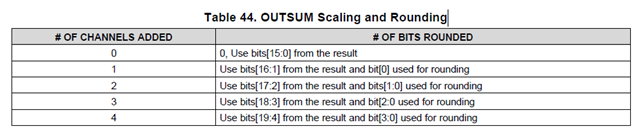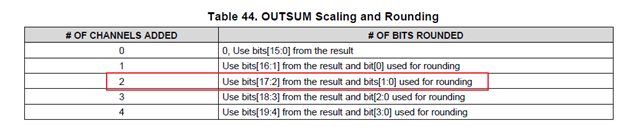# DAC38RF89: Output summation block

Part Number: DAC38RF89

Hi,

In the section 8.3.20 of the datasheet, table 44,the outsum block perform rounding operation after addition.

Why use bits[16:1] from the result when only one channel is added? Isn't it half the result？

I think that there is no overflow when only one channel is added.• User,

This table is with respect to 2's comp data. With two channels added at max value, the result will be a 17 bit number. This is why bits 16:1 are used for this case.

Regards,

Jim

• Hi，Jim

But in table 44, when two channel added, it use bits 17:2  for the result, not bits 16:1. what's the reason?Regards,

John

• John,

With three 16 bit 2's comp numbers at max value added, will result in a 18 bit number. Bits 17:2 will then be used to create the 16 bit value. In this table, you always need to add 1 more channel to the first column.

Regards,

Jim

• Jim,

With three channel added,it will be four 16 bit 2's comp numbers summation, It will result in a 18bit number. But in the table this is a 19 bit number, and it use bits 18:3 for the result.

• [18:3] is 16 bits. Not sure why you say 19 bits.

• Jim,

The sum is bits[18:0] (when three channel added) in the table,it is a 19bit number. But the table use bits 18:3 as result to send to DAC core.

You said "In this table, you always need to add 1 more channel to the first column."

So I think that It's the sum of four 16 bit 2's comp numbers when when three channel added.

Regards,

John.

• User,

You are correct. There is an error in the data sheet. The table should look as shown in the attachment. This will be updated in the next release of the data sheet.

Regards,

Jim